# 2nd PUC Basic Maths Question Bank Chapter 18 Differential Calculus

Students can Download Basic Maths Question Bank Chapter 18 Differential Calculus Questions and Answers, Notes Pdf, 2nd PUC Basic Maths Question Bank with Answers helps you to revise the complete Karnataka State Board Syllabus and to clear all their doubts, score well in final exams.

## Karnataka 2nd PUC Basic Maths Question Bank Chapter 18 Differential Calculus

### 2nd PUC Basic Maths Differential Calculus One Mark Questions and Answers

Question 1.
xe + ex + log a.
Let y = xe + ex + log a
$$\frac{d y}{d x}$$ = exe + ex + 0 = exe + ex.

Question 2.
$$\sqrt{x+1}$$
Let y = $$\sqrt{x+1}$$
$$\frac{d y}{d x}=\frac{1}{2 \sqrt{x+1}}$$Question 3.
xe + ex + ee.
Let y = xe + ex + ee
$$\frac{d y}{d x}$$ = exe-1 + ex + 0
= exe-1 + ex

Question 4.
log (3x + 5).
Let log (3x + 5)
$$\frac{d y}{d x}=\frac{3}{3 x+5}$$

Question 5.
$$\frac{7}{e^{-4 x}}$$
Let y = 7.e4x
$$\frac{d y}{d x}$$ = 7.e4x
= 28e4x

Question 6.
$$\frac{1}{\sqrt{x^{5}}}$$Question 7.
$$\sqrt{x^{4}}$$
Let y = (x4)1/3 = x4/3
$$\frac{d y}{d x}=\frac{4}{3} x^{4 / 3-1}$$
= $$\frac{4}{3} \cdot x^{1 / 3}$$

Question 8.
ex2
Let y = ex2
$$\frac{d y}{d x}$$ = ex2 .2x.

Question 9.
5ex – logx – 3√x.
Let y = 5ex – logx – 3√x
$$\frac{d y}{d x}$$ = 5ex – $$\frac{1}{x}-\frac{3}{2 \sqrt{x}}$$

Question 10.
log (cos x)
Let y = log (cos x)
$$\frac{d y}{d x}$$ = $$\frac{1}{\cos x}$$ . – sin x = -tanx

Question 11.
sin (log x)
Let y = sin (log x)
$$\frac{d y}{d x}$$ = cos(log x) .$$\frac { 1 }{ x }$$
$$=\frac{\cos (\log x)}{x}$$Question 12.
log (Iogx).
Let y = log (log x)
$$\frac{d y}{d x}=\frac{1}{x \cdot \log x}$$

Question 13.
$$e^{\sqrt{x}}$$
Let y = $$e^{\sqrt{x}}$$
$$\frac{d y}{d x}=e^{\sqrt{x}} \cdot \frac{1}{2 \sqrt{x}}$$

Question 14.
$$\sqrt{4 x+7}$$Question 15.
$$\sqrt{\tan x}$$Question 16.
cot3x.
Let y = (cot x)3
$$\frac{d y}{d x}$$ = 3 cot2 x.(-cosec2x)
= – 3 cot2 x. cosec2x.

Question 17.
(2x2 + 4x + 5)5.
Let y = (2x2 + 4x + 5)5
$$\frac{d y}{d x}$$ = 5(2x2 + 4x + 5)4(4x + 4).

Question 18.
tan √x.
Let y = tan √x
$$\frac{d y}{d x}=\frac{\sec ^{2} \sqrt{x}}{2 \sqrt{x}}$$

Question 19.
log (ax + b)
Let y = log (ax + b)
$$\frac{d y}{d x}=\frac{a}{a x+b}$$

Question 20.
e(5x + 6)
Let y = e(5x + 6)
$$\frac{d y}{d x}$$ = 5 . e(5x + 6)Question 21.
log (6 – 5x)
Let y = log (6 – 5x)
$$\frac{d y}{d x}=\frac{-5}{6-5 x}$$

Question 22.
e-3x2
Let y = e-3x2
$$\frac{d y}{d x}$$ = -e-3x2 .6x.

### 2nd PUC Basic Maths Differential Calculus Two Marks Questions and Answers

Question 1.
If x = 5t2 and y = 10t find $$\frac{d y}{d x}$$.
diff both w.r.t rQuestion 2.
If x2 + y2 = a2 find
diff w.r.t x
2x + 2y $$\frac{d y}{d x}$$ = 0 ⇒ $$\frac{d y}{d x}=-\frac{x}{y}$$

Question 3.
If x2 + y2 = 13 find dy/dx when x = 3 and y = -2.
diff w.r.t x
2x + 2y $$\frac{d y}{d x}$$ = 0
$$\frac{d y}{d x}=-\frac{-2 x}{2 y}=\frac{-3}{-2}=\frac{3}{2}$$

Question 4.
If x2 + y2 + 2xy = 13 find $$\frac{d y}{d x}$$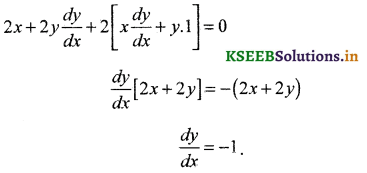Question 5.
Differentiate logx2 3 w.r.t x.
Let y = logx2 3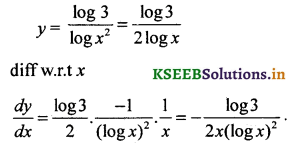Question 6.
If x2y + y2 = 5 find $$\frac{d y}{d x}$$.
diff w.r.t xQuestion 7.
If x = at2 y = 2at find $$\frac{d y}{d x}$$
diff both w. r.t t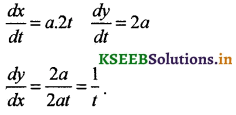Question 8.
If y = xx find $$\frac{d y}{d x}$$
Taking logm both sides
log y = logxx
log y = x logx
diff w.t.r xQuestion 9.
If y = $$(\sqrt{x})^{x}$$Question 10.
If $$\sqrt{x}+\sqrt{y}=\sqrt{a}$$ find $$\frac{d y}{d x}$$ at (1,4)
diff w.r.t x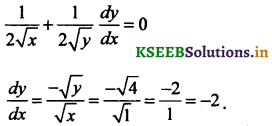Question 11.
If f(x) = x2 – 3x + 10 find f1(50) and f1(11).
diff w.r.t x
f1(x) = 2x – 3
f1(50) = 100 – 3 = 97
f1(11) = 22 – 3 = 19.

Question 12.
If f(x) = xn & If f1 = 10 find n.
f'(x) = n . xn – 1
f1(1) = n(1)n – 1 1
0 =n . 1n – 1 ⇒ n = 10.Question 13.
If y = log(x + 1 + x2) P.T $$\frac{d y}{d x}=\frac{1}{\sqrt{1+x^{2}}}$$
diff w.r.t x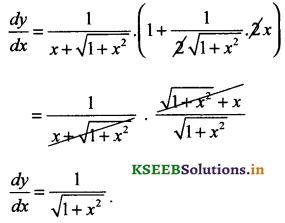Question 14.
If y = $$\frac{\cos x}{1+\sin x}$$ find $$\frac{d y}{d x}$$
diff w.r.t x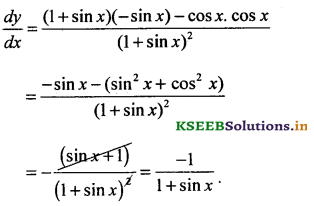Question 15.
y = tan (log (sin x)) find $$\frac{d y}{d x}$$
$$\frac{d y}{d x}$$ = sec2(log(sin x)). $$\frac{\cos x}{\sin x}$$
= sec2(log(sin x)). cot x

Question 16.
If y = cot $$\left(x^{2}+\frac{1}{x^{2}}\right)$$
diff w.r.t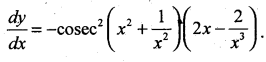Question 17.
If y = log$$\left(\frac{1-x^{2}}{1+x^{2}}\right)$$ find $$\frac{d y}{d x}$$
y = log (1 – x2) – log (1 + x2)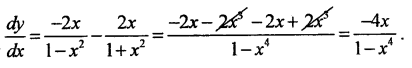Question 18.
y = log$$\left(\frac{1+\sin x}{1-\sin x}\right)$$ find $$\frac{d y}{d x}$$
y = log(1 + sinx) – log (1 – sin x)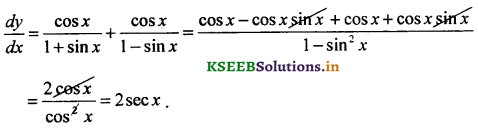### 2nd PUC Basic Maths Differential Calculus Three Marks Questions and Answers

Question 1.
Differentiate ex by 1st Principles.
Let y = ex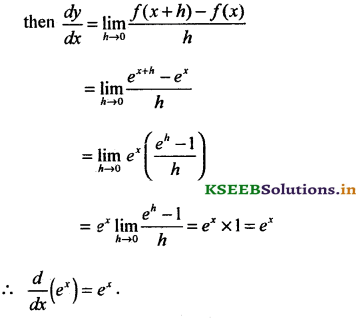Question 2.
If y = ax + y S.T $$\frac{d y}{d x}=\frac{y \log a}{1-y \log a}$$
diff w.r.t xQuestion 3.
If y. eY = x S.T $$\frac{d y}{d x}=\frac{y}{x(y+1)}$$Question 4.
if x = y2 log x S.T $$\frac{d y}{d x}=\frac{y\left(x-y^{2}\right)}{2 x^{2}}$$
diff w.r.t x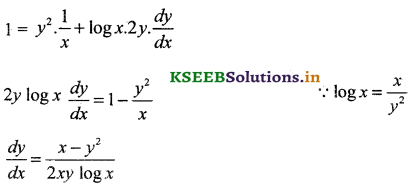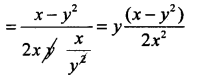Question 5.
If xm. yn = am+n then S.T $$\frac{d y}{d x}=\frac{-m y}{n x}$$
Taking logm bothsides
log (xm . yn) = (a)m+n
Iogxm + log yn = Iog (m+n)Ìoga
diff w.r.t x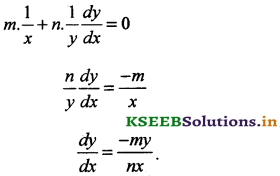Question 6.
If $$\sqrt{x+\sqrt{x+x+}}$$ ……… ∞ P.T $$\frac{d y}{d x}=\frac{1}{2 y-1}$$
y = $$\sqrt{x+y}$$ S.BS
S.B.S
y2 = x + y
diff w.r.t xQuestion 7.
If y = $$\sqrt{\log x+\sqrt{\log x+\log x \ldots . . \infty}}$$ S.T (2y – 1) $$\frac{d y}{d x}=\frac{1}{x}$$
y = $$\sqrt{\log x+y}$$
S.B.S
y2 = log x + y
diff w.r.t xQuestion 8.
Differentiated ax from 1st Principles.
Let y = ax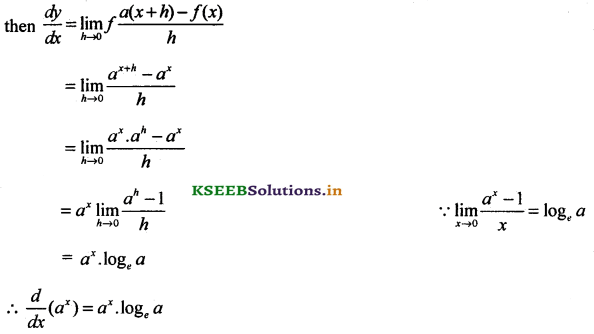Question 9.
Differentiated xa from 1st Principles.
Let y = f(x) = xn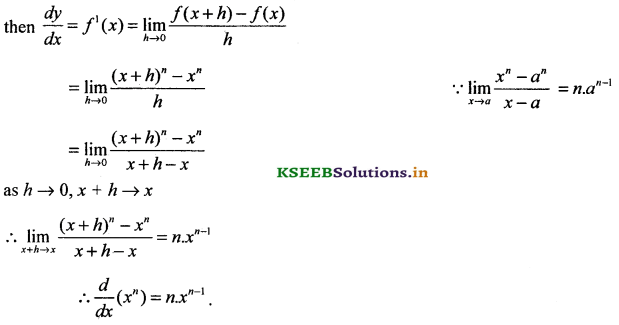Question 10.
If y = $$\sqrt{\frac{1-\cos 2 x}{1+\cos 2 x}}$$ find $$\frac{d y}{d x}$$
y = $$\sqrt{\frac{2 \sin ^{2} x}{2 \cos ^{2} x}}$$
y = tan x
$$\frac{d y}{d x}$$ = sec2

Question 11.
If y = $$(x+\sqrt{x^{2}+1})^{n}$$ P.T (x2 + 1) $$\left(\frac{d y}{d x}\right)^{2}$$ = n2 y2
y = $$(x+\sqrt{x^{2}+1})^{n}$$
diff w.r.t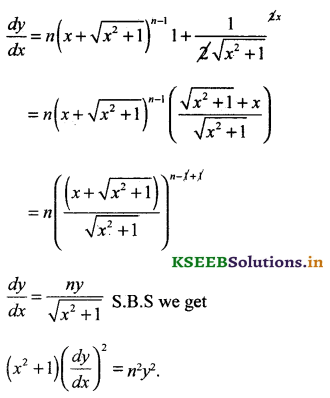Question 12.
If ex + ey = ex+y S.T $$\frac{d y}{d x}$$ = -ey – x
diff w.r.t x
ex + ey $$\frac{d y}{d x}$$ = ex+y$$\left(1+\frac{d y}{d x}\right)$$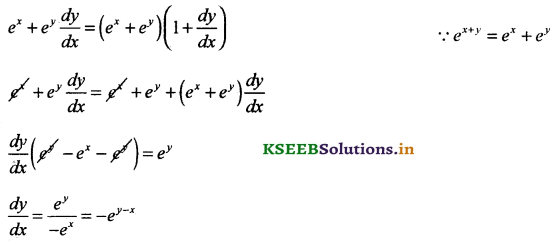Question 13.
If sin y = x sin (a +y) then P.T $$\frac{d y}{d x}=\frac{\sin ^{2}(a+y)}{\sin a}$$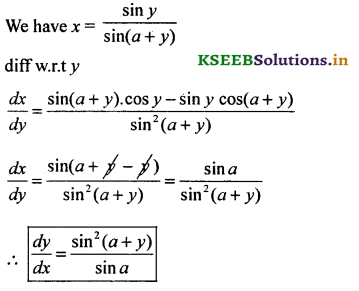Question 14.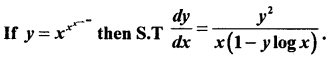We have y = xy apply logm both sides we get
log y = y log x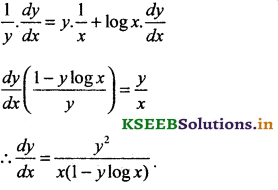Question 15.
If y = (sin x)tanx find $$\frac{d y}{d x}$$.
Taking logm both sides we get
log y = log (sin x)tan x tanx
log y = tan x. log (sin x)
$$\frac{1}{y} \cdot \frac{d y}{d x}=$$ = tan x . $$\frac{\cos x}{\sin x}$$ + log (sin x). sec2x
$$\frac{d y}{d x}$$ = y[1 + sec2x. log(sin x)].

Question 16.
If xy = P.T $$\frac{d y}{d x}=\frac{\log x}{(1+\log x)^{2}}$$
Taking log m both sides
y log x = (x – y)
= x – y ∵ log e = 1
y + y log x = x
y(1 + logx) = x### 2nd PUC Basic Maths Differential Calculus Five Marks Questions and Answers

Question 1.
If $$x \sqrt{1+y}+y \sqrt{1+x}=0$$ = 0 where x ≠ y S.T $$\frac{d y}{d x}=\frac{-1}{(1+x)^{2}}$$
We have $$x \sqrt{1+y}=-y \sqrt{1+x}$$ S.B.S we get
x2(1 + y) = y2(1 + x)
x2 + x2y – y2 – xy2 = 0
(x2 – y2) + (x2y – xy2) = 0
(x – y) (x + y) + xy (x – y) = 0
(x – y)(x + y + xy) = 0 ⇒
x + y + xy = 0 ∵ x ≠ y
diff w.r.t t y(1 + x) = -x ⇒ y = $$\frac{-x}{1+x}$$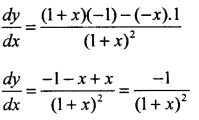Question 2.
If y = ex logx S.T xy2 – (2x – 1)y + (x – 1)y = 0.
diff w.r.t x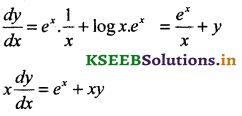diff again w.r.t x d2y dy
x $$\frac{d^{2} y}{d x^{2}}+\frac{d y}{d x}$$ . 1 = ex + x. $$\frac{d y}{d x}$$ + y .1 ∵ e = xy1 – xy
xy2 + y1 – xy1 -xy + xy1 + y1 = o
xy2 + y1 – 2xy1 + xy – y = 0
xy2 – (2x – 1) y1 + (x – 1)y = 0.

Question 3.
If y = $$(x+\sqrt{1+x^{2}})^{m}$$ P.T (1 + x2)y2 + xy1 – m2y = 0
diff w.r.t x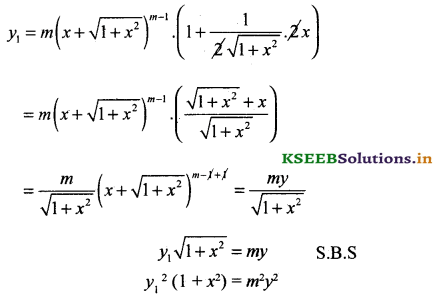Again diff w.r.t x
(1 + x2)2y1 y2 + y21 (2x) = m2.2y.y1 (divide by 2y1)
(1 + x2)y2 + xy1 – m2y = 0.Question 4.
If y = $$x+\sqrt{x^{2}-1}$$ S.T (x2 – 1)y2 + xy1 – y = 0
diff w.r.t x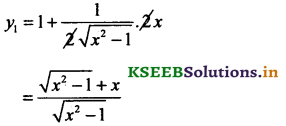$$\sqrt{x^{2}-1}$$, y1 = y S.B.S
(x2 – 1)y12 = y2
(x2 – 1)2y1y1 . 2x = 2yy1 + by 2y1 we get
(x2 – 1)y2 + xy1 – y = 0.

Question 5.
If xy + 4y = 3x S.T $$\frac{d^{2} y}{d x^{2}}=\frac{-2 y}{(x+4)^{3}}$$
y(x + 4) = 3xQuestion 6.
Ify = (x2 + a2)5 S.T (x2 – a2) $$\frac{d^{2} y}{d x^{2}}$$ – 10x $$\frac{d y}{d x}$$ – 12y = 0
diff w.r.t x
$$\frac{d y}{d x}$$ = 6(x2 + a2)5 . 2x multiply (x2 + a2)
(x2 + a2)$$\frac{d y}{d x}$$ = 12(x2 + a2)
(x2 + a2)$$\frac{d y}{d x}$$ = 12xyQuestion 7.
If x = at2 & y = 2at find $$\frac{d^{2} y}{d x^{2}}$$
diff w.r.t xQuestion 8.
If y = a xn + 1 + $$\frac{b}{x^{n}}$$ P.T. x2$$\frac{d^{2} y}{d x^{2}}$$ = n(n + 1)y .
y = a.xn + 1 + b.x-n
diff w.r.t x
y1 = a(n + 1)xn + b – n. x-n-1
y1 = a{n + 1)xn – bn. xn – 1
Again diff w.r.t x
y2 = a(n + 1) n.xn-1 – b.n(-n – 1) x-n-2
y2 = n(n + 1) [axn-1 + bx-n-2] multiply both sides by x2
x2y2 = n(n + 1) (a.xn – 1 . x2 + b.x -n-2. x2)
= n(n + 1) a.xn-1 + b.x-n
x2y2 = n(n + 1 ).yQuestion 9.
If 4x2 + 9y2 = 36 P.T = $$\frac{d^{2} y}{d x^{2}}=\frac{-16}{9 y^{3}}$$
diff w.r.t x
8x + 18y.y1 = 0
y1 = $$\frac{-8 x}{18 y}=\frac{-4 x}{9 y}$$ ______ (1)
Again diff w.r.t xQuestion 10.
If xmyn = (x + y)m+n S.T $$\frac{d y}{d x}=\frac{y}{x}$$
Taking logm both sides we get
log (xm.yn) = log (x + y)m+n</sup
m log x + n log y = (m + n) log (x + y)
diff w.r.t x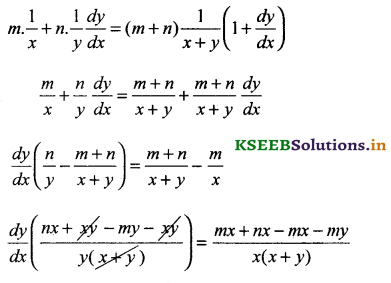Question 11.
If y2 + 2y = x2 S.T y1 = $$\frac{1}{(1+y)^{3}}$$
diff w.r.t x
2yy1 + 2y1 = 2x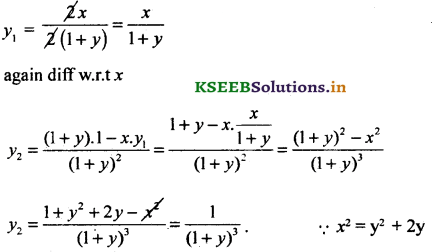Question 12.
If y = 3e2x + 2.e3x P.T y2 – 5y1 + 6y = 0.
diff w.r.t x
y1 = 6e2x + 6.e3x
y1 = 6(e2x + e3x)
y2 = 6(2e2x + 3e3x)
Consider LH.S = y2 – 5y1 + 6y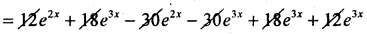= 0 = R.H.S.

Question 13.
If y = a cos (log x) + b sin(logx) S.T x2y2 + xy1 +y = 0.
diff w.r.t x
y1 = a$$\left[\frac{-\sin (\log x)}{x}\right]+b\left[\frac{\cos (\log x)}{x}\right]$$
xy1 = -a sin (Iogx) + b cos(1ogx)
diff again w.r.t x
xy2 + y1 = $$-\frac{a \cos (\log x)}{x}-\frac{b \sin (\log x)}{x}$$
x2y2 + xy1 = -(a cos log x + b sin(1ogx)
x2y2 + x1 + y = 0.

Question 14.
If y = sin (logx) S.T x2y2 + xy1 + y = 0.
diff w.r.t x
y1 = $$\frac{\cos (\log x)}{x}$$
xy1 = cos(logx)
duff again w.r.t x
x.y2 + y1 . 1 = $$\frac{-\sin (\log x)}{x}$$
⇒ x2y2 + xy1 = 0Question 15.
Differentiate cotx from Ist Principles.
Let y = f(x) = cot tQuestion 16.
If y = xlogx + (log x)x find $$\frac{d y}{d x}$$
Let y = u + v
$$\frac{d y}{d x}=\frac{d u}{d x}+\frac{d v}{d x}$$ ______ (1)
where u = xlogx and V = (log x)x
Taking logm both sides
log u = log x. log x,
log u = (log x)2
diff both w.r.tx logV = x . log (log x)Question 17.
If x = a[cos t + log tan $$\frac { t }{ 2 }$$] y = a sin t, S.T $$\frac{d y}{d x}$$ = tan t.